Study Materials: ncert solutions

Our ncert solutions for Exercise 7.2 Class 9 maths 7. Triangles - ncert solutions - Toppers Study is the best material for English Medium students cbse board and other state boards students.

Solutions ⇒ Class 9th ⇒ Mathematics ⇒ 7. Triangles

# Exercise 7.2 Class 9 maths 7. Triangles - ncert solutions - Toppers Study

Topper Study classes prepares ncert solutions on practical base problems and comes out with the best result that helps the students and teachers as well as tutors and so many ecademic coaching classes that they need in practical life. Our ncert solutions for Exercise 7.2 Class 9 maths 7. Triangles - ncert solutions - Toppers Study is the best material for English Medium students cbse board and other state boards students.

## Exercise 7.2 Class 9 maths 7. Triangles - ncert solutions - Toppers Study

CBSE board students who preparing for class 9 ncert solutions maths and Mathematics solved exercise chapter 7. Triangles available and this helps in upcoming exams 2023-2024.

### You can Find Mathematics solution Class 9 Chapter 7. Triangles

• All Chapter review quick revision notes for chapter 7. Triangles Class 9
• NCERT Solutions And Textual questions Answers Class 9 Mathematics
• Extra NCERT Book questions Answers Class 9 Mathematics
• Importatnt key points with additional Assignment and questions bank solved.

NCERT Solutions do not only help you to cover your syllabus but also will give to textual support in exams 2023-2024 to complete exercise 7.2 maths class 9 chapter 7 in english medium. So revise and practice these all cbse study materials like class 9 maths chapter 7.2 in english ncert book. Also ensure to repractice all syllabus within time or before board exams for ncert class 9 maths ex 7.2 in english.

See all solutions for class 9 maths chapter 7 exercise 7 in english medium solved questions with answers.

### Exercise 7.2 class 9 Mathematics Chapter 7. Triangles

• Exercise 7.2 Class 9 Maths 7. Triangles - Ncert Solutions - Toppers Study
• Class 9 Ncert Solutions
• Solution Chapter 7. Triangles Class 9
• Solutions Class 9
• Chapter 7. Triangles Exercise 7.2 Class 9

## Exercise 7.2 Class 9 maths 7. Triangles - ncert solutions - Toppers Study

Chapter 7. Triangles

Exercise 7.2

Q1. In an isosceles triangle ABC, with AB = AC, the bisectors of ∠ B and ∠ C intersect each other at O. Join A to O. Show that :

(i) OB = OC (ii) AO bisects ∠ A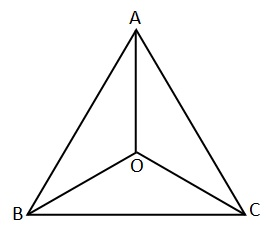Solution:

Given: In an isosceles triangle ABC, with AB = AC,  the bisectors of ∠ B and ∠ C intersect each other at O.

To prove:

(i) OB = OC

(ii) AO bisects ∠ A

Proof: In  ΔABC, We have:

AB = AC

∠ B = ∠ C [ opposite angle to the equal side ]

Or  1/2 ∠ B = 1/2 ∠C

So, ∠OBC = ∠OCB […1]

In D ABO and D ACO

AB = AC [given]

∠OBC = ∠OCB [from …1]

AO = AO [common]

By SAS Congruence Criterion Rule

DABO  DACO

OB = OC [ By CPCT ]

∠BAO = ∠CAO [ By CPCT ]

AO bisect ∠A.

Q2. In Δ ABC, AD is the perpendicular bisector of BC (see Fig. 7.30). Show that Δ ABC is an isosceles triangle in which AB = AC.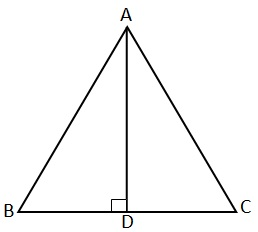Solution:

Given: In Δ ABC, AD is the perpendicular bisector of BC.

To prove: Δ ABC is an isosceles triangle in which AB = AC.

Proof: In Δ ABD and Δ ACD, we have

DB = DC [since D bisect BC]

By SAS Congruence Criterion Rule

Δ ABD  Δ ACD

AB =AC [CPCT]

Hence, Δ ABC is an isosceles triangle

Q3. ABC is an isosceles triangle in which altitudes BE and CF are drawn to equal sides AC and AB respectively (see Fig. 7.31). Show that these altitudes are equal.

Solution:

Given: ABC is an isosceles triangle in which BE ⊥ AC and CF ⊥ AB where AB = AC.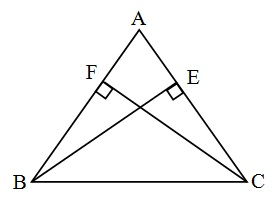To prove: BE = CF.

Proof:  Here, BE ⊥ AC and CF ⊥ AB  (Given)

In ΔABE and Δ ACF

∠ AEB = ∠ AFC  (90 Each)

∠ A = ∠ A       (Common)

AB = AC        (Given)

By ASA Congruency Criterion Rule

ΔABE    Δ ACF

BE = CF [ By CPCT ]

Q4. ABC is a triangle in which altitudes BE and CF to sides AC and AB are equal (see Fig. 7.32). Show that

(i) Δ ABE ≅ Δ ACF

(ii) AB = AC, i.e., ABC is an isosceles triangle.Solution:

Given: ABC is a triangle in which

BE ⊥ AC and CF ⊥ AB and BE = CF

To Prove :

(i) Δ ABE ≅ Δ ACF

(ii) AB = AC, i.e., ABC is an isosceles triangle.

Proof :

(i) In Δ ABE and Δ ACF

BE = CF           (Given)

∠ AEB = ∠ AFC     (90 Each)

∠ A = ∠ A         (Common)

Using ASA Congruency Property

Δ ABE ≅ Δ ACF   Proved I

(ii)            AB = AC  [By CPCT

Therefore, ABC is an isosceles triangle.

Q5. ABC and DBC are two isosceles triangles on the same base BC (see Fig. 7.33). Show that ∠ ABD = ∠ ACD.

Solution: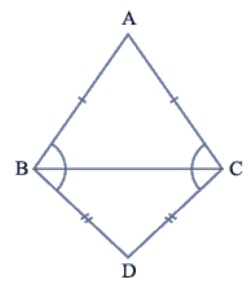Given : ABC and DBC are two isosceles triangles on the same base BC.

To prove : ∠ ABD = ∠ ACD

Proof : ABC is an isosceles triangle in which

AB = AC      (Given)

∴      ∠ ABC = ∠ ACB           .......... (1)

(Opposite angles of equal sides)

Similarily,

BCD is also an isosceles triangle.

BD = CD      (Given)

∴      ∠ DBC = ∠ DCB           .......... (2)

(Opposite angles of equal sides)

∠ ABC + ∠ DBC = ∠ ACB + ∠ DCB

Or,           ∠ ABD = ∠ ACD       Proved

Q6. ΔABC is an isosceles triangle in which AB = AC. Side BA is produced to D such that AD = AB (see Fig. 7.34). Show that ∠ BCD is a right angle.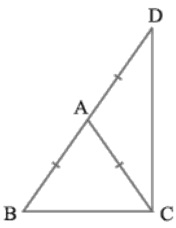Solution :

Given: ΔABC is an isosceles triangle in which AB = AC.

Side BA is produced to D such that AD = AB

To prove : ∠ BCD = 90

Proof :

AB = AC      .............. (1)  (Given)

And,   AB = AD      .............. (2)  (Given)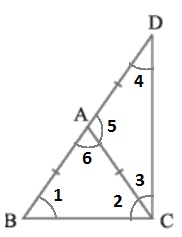From equation (1) and (2) we have

∴      ∠3 = ∠4     .... (4) (Opposite angles of equal sides)

Now, AB = AC    from (1)

∴       ∠1 = ∠2     .... (5) (Opposite angles of equal sides)

In ΔABC,

Exterior ∠5 = ∠1 + ∠2

Or,         ∠5 = ∠2 + ∠2       from (5)

Or,         ∠5 = 2∠2    ....... (6)

Similarily,

Exterior ∠6 = ∠3 + ∠4

Or,         ∠6 = 2∠3      from (7)

∠5 + ∠6  = 2∠2 + 2∠3

∠5 + ∠6  = 2(∠2 + ∠3)

Or,     180० = 2(∠2 + ∠3)   [ ∵ ∠BAC + ∠DAC  = 180० ]

Or,    ∠2 + ∠3 = 180० / 2

Or,     ∠BCD = 90०      Proved

Q7. ABC is a right angled triangle in which ∠ A = 90° and AB = AC. Find ∠ B and ∠ C.

Solution: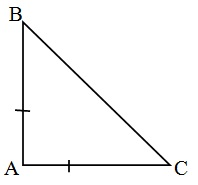Given : ABC is a right angled triangle in which

∠ A = 90° and AB = AC.

To Find : ∠B and C

AB = AC          (Given)

∴            ∠B = ∠C      ............(1)

(Opposite angles of equal sides)

In triangle ABC,

∠A + ∠B + ∠C = 180०   (Angle sum property)

90° + ∠B + ∠B = 180०   Using equation (1)

∠B = 180- 90°

2 ∠B = 90°

∠B =  90°/ 2

∠B =  45°

∴ ∠B = 45° and ∠C =  45°

Q8. Show that the angles of an equilateral triangle are 60° each. ⊥

Solution: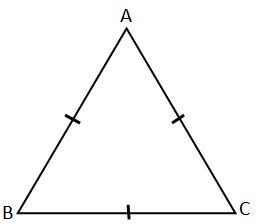Given: ABC is a equilateral triangle, in which

AB = BC = AC

To Prove :

∠A = ∠B = ∠C = 60°

Proof :

AB = AC      (Given)

∠B = ∠C     ....................... (1)   [opposite angle of equal sides]

AB = BC       (Given)

∠A = ∠C     ....................... (2)   [opposite angle of equal sides]

AC = BC       (Given)

∠A = ∠B     ....................... (3)   [opposite angle of equal sides]

From equation (1), (2) and (3) we have

∠A = ∠B = ∠C     .............. (4)

In triangle ABC

∠A + ∠B + ∠C = 180°

∠A + ∠A + ∠A = 180°

3 ∠A = 180°

∠A = 180°/3

∠A = 60°

∴ ∠A = ∠B = ∠C = 60°

##### Other Pages of this Chapter: 7. Triangles

Important Study materials for classes 06, 07, 08,09,10, 11 and 12. Like CBSE Notes, Notes for Science, Notes for maths, Notes for Social Science, Notes for Accountancy, Notes for Economics, Notes for political Science, Noes for History, Notes For Bussiness Study, Physical Educations, Sample Papers, Test Papers, Mock Test Papers, Support Materials and Books.Mathematics Class - 11th

NCERT Maths book for CBSE Students.

books

## Study Materials List:

##### Solutions ⇒ Class 9th ⇒ Mathematics
1. Number Systems
2. Polynomials
3. Coordinate Geometry
4. Linear Equation In Two Variables
5. Introduction To Euclid’s Geometry
6. Lines and Angles
7. Triangles